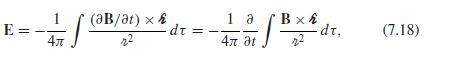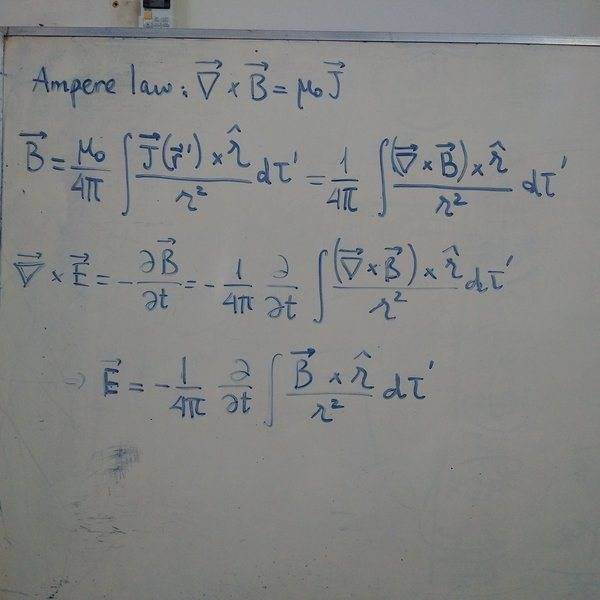# Analog to Biot-Savart for E field

• I
scoomer
On page 317 of "INTRODUCTION TO ELECTRODYNAMICS" 4th Ed. by Griffiths he states without proof that the analog to Biot-Savart for finding the E field is:Can anyone direct me to a reference where this is proved or give me a hint how to prove it? Thank you.

#### Attachments

Nguyen Son
Hope it can help you#### Attachments

•scoomer
##\nabla \cdot E=\frac{1}{\epsilon}\rho##
Gauss's law ##E=\frac{1}{4\pi\epsilon}\int \frac{(\nabla \cdot E) \hat r}{r^2} d \tau##
Use the identity ##A \times (B \times C) = B (A \cdot C)-C(A\cdot B)## where ##A=\nabla##, and possibly integrate by parts, I am not sure,
You get an an integral like ##\frac{1}{4\pi}\int \frac{(\nabla \times E) \times \hat r}{r^2} d\tau##.
Use Maxwell's equation ##\nabla \times E = -\frac {\partial B}{\partial t}##.
This gives you ##E=-\frac {1}{4\pi} \int \frac {(\frac {\partial B}{\partial t}) \times \hat r}{r^2} d\tau##
Since you are integrating over space and not time, we can move the partial derivative out of the integral, getting
##E=-\frac {1}{4\pi} \frac{\partial}{\partial t} \int \frac { B \times \hat r}{r^2} d\tau##
I hope this helps.

•scoomer
Mentor
On page 317 of "INTRODUCTION TO ELECTRODYNAMICS" 4th Ed. by Griffiths
I don't have the 4th edition. In the 3rd edition, your equation appears as part of Problem 7.47:
Use the analogy between Faraday's Law and Ampère's law, together with the Biot-Savart law, to show that <<your equation>> for Faraday-induced electric fields.
So if you assume the validity of Biot-Savart, you can start from there and use the similarity between Faraday and Ampère to suss out the analogous equation for ##\vec E##. Or you can look for a derivation of Biot-Savart from Maxwell's equations, and then adapt that to work for ##\vec E## instead of ##\vec B##, again using the similarities between the various Maxwell equations.

•scoomer and BvU
scoomer